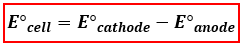# Problem: Given that E°cell = 3.20V for the reaction 2Na(in Hg) + Cl2(g) ⇌ 2Na+(aq) + 2Cl−(aq)What is E° for the reduction 2Na+(aq) + 2e−→ 2Na(in Hg)?

###### FREE Expert Solution

We’re being asked to determine the standard reduction potential (E°red) of Na+/Na (inHg) from a reaction occurring in an electrochemical cell. To calculate for the standard reduction potential, use the following equation:When a balanced reaction is given, identify the anode and the cathode half-reactions by determining the changes in oxidation states of each species based on the given reaction:

Recall:

82% (425 ratings)###### Problem Details

Given that E°cell = 3.20V for the reaction 2Na(in Hg) + Cl2(g) ⇌ 2Na+(aq) + 2Cl(aq)

What is E° for the reduction 2Na+(aq) + 2e→ 2Na(in Hg)?

Frequently Asked Questions

What scientific concept do you need to know in order to solve this problem?

Our tutors have indicated that to solve this problem you will need to apply the Cell Potential concept. If you need more Cell Potential practice, you can also practice Cell Potential practice problems.